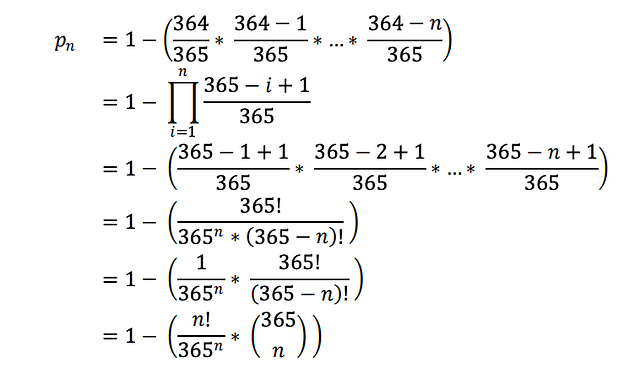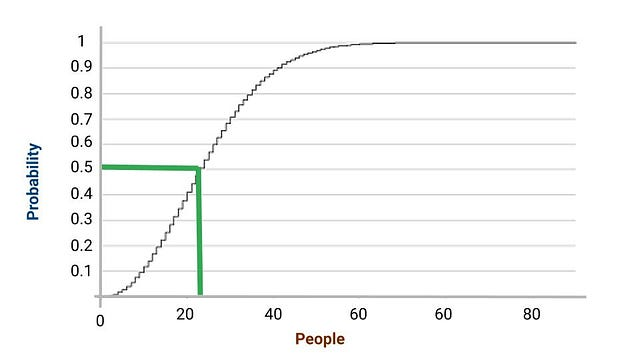The birthday paradox is strange and counter-intuitive. It's a "paradox" because our brain finds it difficult to handle the compounding power of exponents. Real-world applications for this include a cryptographic attack called the "birthday attack".

Birthday Paradox is one of the most interesting but also challenging to understand and explain to others! Moreover, there is a similar problem that seems to be equivalent, but in fact, it is not. Therefore, we will concentrate on this difference and show it using a simple example.

### Problem Statement

What is the probability that at least two of them have the same birthday in a given set of n randomly chosen people? A more specific version but the same problem is: How many people must be in a group of randomly chosen people, such that there is more than 50% probability that at least two of them have the same birthday.

We will first try to solve the specific version of the problem before deriving the general formula. Suppose the number of people in the group is n and a year has 365 days.

Case 1: For n = 1

There is one person in the group and that person has definitely a birthday on one day in the year. So probability p = 1 = 365/365.

Case 2: For n = 2

The group consists of two people. Person1 has a birthday one day in a year. Then the probability that person2 has a birthday on another day than person1 is 364/365 (there are 364 remaining days). So the probability that both persons have the same birthday p = 1 - 364/365 = 1/365.

Case 3: For n = 3

Here we extend the case n = 2. Again, person 1 has a birthday on one day, and person 2 has a birthday on a different day with p = 364/365. So it follows that person 3 has a birthday on a different day than person 1 and person 2 with a probability of 363/365.

• So the probability that three people have different birthdays p' = 1 * (364/365) * (363/365) ≈ 99.1796%.
• So the probability that at least two of them have the same birthday p = 1 – p' = 1 – (1 * 364/365 * 363/365) ≈ 0.0082%

So we can derive a general formula for n persons.If we put n = 23 in the above formula, the probability is 50.7%. That means in a room with 23 people, the probability that two or more persons have the same birthday is bigger than 50%! In a room with 41 people, it‟s even bigger than 90%!In general, suppose there are k people and n dates. The simple way to approach this problem is to start by finding the probability of not getting a match. Imagine you are putting the names of people into some boxes one by one. The first name would have no matches. The second name would have a choice of n-1 out of n names to avoid a match, or 1 – 1/n. The third name would have a choice of n-2 out of n names to prevent a match or 1 – 2/n. If we were to continue this trend for k people:

P (Chance of no matche) = (1 − 1/n) (1 − 2/n) … (1 − k-1/n)

= (n - 1)(n - 2)...(n - k - 1)/n^k

= !n/(n^k)(!n-k)

P (Chances of a match) = 1 - P (Chance of no matche)

For n much larger than k, each factor is close to 1. This corresponds to a low chance of matching for each factor. This formula could solve the problem, given k and n.

### The Intuitive Variation in the Birthday Paradox

For most of us, this result seems to be surprisingly low. We would have estimated many more people to exceed the 50% border. Other estimated even 365/2 = 182. The most obvious reason is that the actual problem is misinterpreted.

Many people mix the birthday paradox with the following question, which is NOT the same: What is the probability that in a given set of n randomly chosen people, at least one of them has the same birthday as a specific person (or at least one of them has a birthday on a particular date)?

Another (similar) version is:

How many people must a group of randomly chosen people contain, such that there is more than a 50% probability that at least one of them has a birthday on the same day as person x?

Compare those versions with the correct birthday paradox to understand the slight but far-reaching difference! So let's solve this one. We want to derive the probability for n people that at least two of them have a birthday on the same predefined date.

Case 1: For n = 1

The first person has a birthday on one date. The probability that this person has no birthday on that date is p' = 364/365. On the other way, the probability that he/she has a birthday on that given date is p = 1 – p' = 1 - 364/365 = 1/365.

Case 2: For n = 2

The first person has no birthday on that date with p' = 364/365. The same applies to the second person. This results that both persons have no birthday on a given date with probability p‟ = p' * p' = (364/365)^2. So the desired probability that at least one of them has a birthday on that date is p = 1 – p‟ = 1 - (364/365)^2.

So we can generalize the formula for n: P = 1 − (364/365)^n.

So for what value of n does this probability exceeds 50%? That is for n >= 253! So there must be at least 253 persons in a room so the probability exceeds 50% that at least two of them have a birthday on a predefined date.

### Critical ideas to think about!

1. We are transforming the birthday paradox into a dice game. Suppose there is n number of people. We assume that each person throws a dice exactly once i.e. they can throw a number between 1 and 6 using a dice once.

• What is the probability that in a given set of n randomly chosen people, at least two of them throw the same number?
• What is the probability that in a given set of n randomly chosen people, a person throws the same number as the first person?
2. For an n-bit hash function, there are 2n possible outputs. Determine the number of outputs t so that among those t outputs, the probability of a collision is greater than 0.5. Note: birthday Paradox is generally discussed with hashing to show the importance of collision handling, even for a small set of keys.

Enjoy learning, enjoy mathematics!